Linear Systems

Solving a Linear System

Given the the Linear System:
ax + by = c
dx + ey = f

For a linear system we have two matrixes as input data:

1 - The Matrix of the Coefficients or Matrix A
[ [a b] [d e] ]

2 - the Matrix of Independent Terms or Matrix B
[ [ c ] [ f ] ]

In HP48/49 we write a matrix as:
[ [ row1] [row2] [ row3] ....[rown]]
and to solve the linear system we can follow the steps below,

 Example 1:  Solve the Linear System    2x+3y=2   5x+6y=9 Example 2:   Solve the Linear System    2x+3y+4z=2   5x+2y+3z=3  5x+6y+3z=-9 PressSOLVE  to access the Solve Lin Sys aplication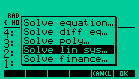PressSOLVE  to access the Solve Lin Sys aplicationEnter the matrixes as shown in the picture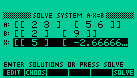Enter the matrixes as shown in the picture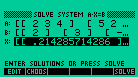Set the browswer bar on the field X and press SOLVE Result  x=5   y=-2.66666666667 Set the browswer bar on the field X and press SOLVE Result  x=.2142854174286  y=-3  z=2.64285714286

 You can also use the Equation Writer to write the matrixes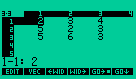Write the Matrix A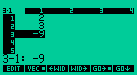Write the Matrix B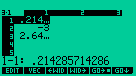and see the result

Solving a Linear System of Complex Numbers

 Lets solve the linear system (5+j3)x + 7j y = 8 j6x + (7-j3) x = 3In HP48/49 syntax we write: (5,3) (0,7) (0,6) (7,-3)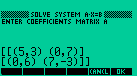Pressand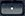to see the entrance in full screen. Enter the data for independent terms and press SOLVE, in the menu.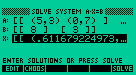Press ENTER to see the result on the stack. Press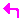EDIT to best see the result.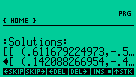It has become appallingly clear that our technology has surpassed our humanity. - Albert Einstein